# Formation of seasonal groups and application of seasonal indices

## Abstract

Estimating seasonal variations in demand is a challenging task faced by many organisations. There may be many stock-keeping units (SKUs) to forecast, but often data histories are short, with very few complete seasonal cycles. It has been suggested in the literature that group seasonal indices (GSI) methods should be used to take advantage of information on similar SKUs. This paper addresses two research questions: (1) how should groups be formed in order to use the GSI methods? and (2) when should the GSI methods and the individual seasonal indices (ISI) method be used? Theoretical results are presented, showing that seasonal grouping and forecasting may be unified, based on a Mean Square Error criterion, and K-means clustering. A heuristic K-means method is presented, which is competitive with the Average Linkage method. It offers a viable alternative to a company's own grouping method or may be used with confidence if a company lacks a grouping method. The paper gives empirical findings that confirm earlier theoretical results that greater accuracy may be obtained by employing a rule that assigns the GSI method to some SKUs and the ISI method to the remainder.

## 1. Introduction

The estimation of seasonal demand patterns is often a challenging task. For some organisations, it is a task that must be accomplished for many hundreds or thousands of stock-keeping units (SKUs). Demand histories may be short for these items, with few complete seasonal cycles. As product life cycles shrink, such short data histories are becoming increasingly common. Consequently, making accurate seasonal estimates for individual SKUs is becoming much more difficult for many products. The implications of inaccurate forecasts may be severe, with detrimental effects on service levels and stock-holdings, ultimately leading to obsolescent stock if remedial action is not taken.

As complete seasonal cycles for individual SKUs may be few, but SKUs themselves may be common, there is an opportunity to take advantage of the abundance of time series by pooling data from many SKUs to estimate seasonal patterns. This idea is not new. Duncan et al (1993) suggested that the use of information on similar time series should benefit forecasting accuracy for a particular series. This argument seems plausible, and reflects the practice in many organisations where seasonality is calculated not at the individual item level, but at the product group level. Similarly, seasonality at local level is often estimated using data at a regional or national level. This practice of seasonal grouping by product or location prompts some questions. How similar must seasonal profiles be for products to benefit from seasonal grouping? Is it always advantageous to group if the products have homogeneous profiles?

Ouwehand et al (2005) commented on the few publications dealing with aggregation approaches to forecasting (some of the most important are those by Dalhart, 1974; Withycombe, 1989; Bunn and Vassilopoulos, 1993, 1999; and Dekker et al, 2004). In this paper, we begin by reviewing work on group seasonal indices (GSI), and find that there are many issues still to be resolved. (For a comprehensive review of recent developments in the area of inventory forecasting in general the interested reader may refer to Syntetos et al, 2009. Chen and Boylan, 2008, provide a review of the literature on seasonal forecasting in particular.)

We identify a selection of unresolved issues in seasonal aggregation, and present results on grouping and forecasting based on the simplest seasonal models. These models, simple as they are, offer insights that may be helpful as research moves on to more complex seasonal models.

## 2. GSI methods

Two main approaches to GSI have been proposed in the literature: one by Dalhart (1974) and the other by Withycombe (1989).

Withycombe (1989) assumed that whatever causes the seasonal fluctuation in demand operates the same on all products within the line (author’s own emphasis). Dalhart (1974) made the same assumption that all subaggregate series had a consistent underlying seasonal behaviour. This assumption led both authors to believe that estimating seasonal indices from the group would be better than from the individual series.

Dalhart (1974) proposed a group seasonal estimation method by averaging the individual seasonal indices (ISI). Let S i =[ai1, ai2,…, a iq ], where a ih is the ISI for item i at season h, S i is the multiplicative seasonal index vector for item i and q is the length of the seasonal cycle. Then S DGSI =1/mi=1mS i where S DGSI is the group seasonal vector of indices estimated by Dalhart’s Group Seasonal Index (DGSI) method and m is the number of series in the group. Therefore, Dalhart’s method is a simple average of the ISI.

Withycombe (1989) proposed a different method to obtain GSI, known as Withycombe’s Group Seasonal Index (WGSI). He totalled all the series in the group and then estimated combined seasonal indices from this single time series. Therefore, Withycombe’s method is a weighted average of the ISI.

While there have been many papers published on seasonal forecasting, there are fewer on aggregation approaches to seasonal forecasting. Rose (1977) investigated the properties of aggregated independent Auto-Regressive Integrated Moving Average (ARIMA) processes, including processes with seasonality. Kim and Moosa (2005) applied seasonal ARIMA models to forecasting international tourist flows to Australia. They found that indirect forecasting of aggregate flows was more accurate than direct forecasting of aggregates. They obtained similar results using regression-based models and structural time series models (Harvey, 1989).

An alternative approach, based on exponential smoothing, was presented by Dekker et al (2004). They proposed that the Holt-Winters method should be adapted, allowing seasonal estimates at the group level while the level and trend estimates remain at the individual level. The researchers applied the new method to the forecasting of sales at two wholesalers (food and electrochemical products) and found the new method to give better performance than the classical Holt-Winters method.

As summarised above, research in this area has progressed by an examination of alternative methods for GSI, with more complex models being addressed as the forecasting field has progressed. However, the fundamental properties of weighted (WGSI) and unweighted (DGSI) seasonal indices, and their forecasting accuracy relative to ISI, have been somewhat neglected. Chen and Boylan (2007) derived rules for the selection of ISI, DGSI and WGSI for an individual series, based on minimisation of the Mean Squared Error (MSE). These rules were subsequently compared with other guidelines (Miller and Williams, 2003) but no results were presented comparing the rules with universal application of seasonal methods.

## 3. Research questions

In the situation outlined in the introduction, an organisation may have hundreds or thousands of SKUs, each with a short demand history, perhaps covering no more than three complete seasonal cycles. If a seasonal grouping approach is to be used, there are some immediate questions.

First, how should the groups be composed? Both Dalhart (1974) and Withycombe (1989) assumed such groups were given, in order to calculate their proposed methods. However, both authors identified the importance of forming seasonally homogeneous groups. More recent work, including that of the present authors, has maintained this assumption, thereby limiting the scope of analysis.

Second, should the grouping method be used for all series in the group, or would it be better to forecast demand for some SKUs using individual seasonal profiles? This question has been addressed theoretically (Chen and Boylan, 2007) but empirical evidence is lacking. This paper will address both research questions.

## 4. Models for the composition of seasonal groups

The composition of seasonal groups has long been recognised as an important question but progress in this area has been slow. Bunn and Vassilopoulos (1993) used cluster analysis with Euclidean distances to define seasonal groups. The software package SPSS/PC+ was used and the average linkage between-groups method was implemented to join clusters and the Euclidean distance to measure nearness (Vassilopoulos, 1994). Although their chosen method resulted in seasonally homogeneous and distinct groups, there was no theoretical justification (such as that provided later in this paper for another clustering approach: K-means). The average linkage method was chosen without consideration or evaluation of any alternative clustering methods.

In order to derive theoretically how seasonally homogeneous groups should be defined, we assume the following two simple models for a number of items m: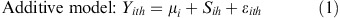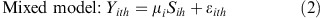where

i :

is a suffix representing the SKU or the location (i=1,…, m)

suffix t:

represents the year and t=1,…, r (where r is the number of years’ data history)

suffix h:

represents the seasonal period and h=1,…, q (where q is the length of the seasonal cycle)

Y :

represents demand

μ i :

represents the underlying mean for the ith SKU or location and is assumed to be constant over time but different for different SKUs or locations

S ih :

represents a seasonal index at seasonal period h; it is unchanging from year to year

ɛ ith :

is a random disturbance term for the ith SKU/location at the tth year and hth period; it is assumed to be identically and independently distributed with mean zero and constant variance σ i 2. It is assumed there is no cross or serial correlation, but this can be relaxed in future research. Under the assumption of no cross correlation, the variance of aggregated demand is σ A 2, which equals i=1mσ i 2.

As discussed in Section 2, more complex models, such as seasonal ARIMA or state-space models (Harvey, 1989), are feasible. Such models are not adopted in this paper, as the aim is to understand the properties of seasonal aggregation methods for the simplest models. It is intended to build on this base in future work, extending the analysis to other models.

## 5. Seasonal grouping for the additive model

Chen and Boylan (2007) showed that, for the additive model, the MSE by using ISI for the ith SKU/location and hth period (MSE ih ) is:Using a similar argument to Chen and Boylan (2007), the MSE for the ith SKU/location, for the hth season, based on a seasonal index for the whole number of SKUs/locations (m) MSE ih is (see Appendix A):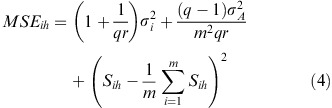where m represents the number of items and σ A 2 represents the (constant) variance of the random disturbance term for the aggregate series (ie aggregated over all items).

Equation (4) shows that if a GSI method is used, the MSE of a particular item depends on the noisiness of the series itself, the noisiness of the aggregated series and a distance metric that measures how close the individual item's seasonality is from the average of the group.

The term (1+1/qr)σ i 2 is not affected by how items are grouped, but the other two terms are affected. Group composition affects σ A 2 as it is the sum of all individual σ i 2s when all items are independent. If cross-correlations are allowed, then σ A 2 is affected by how the correlation matrix is defined. The magnitude of the termis also affected.

The termor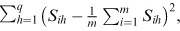when summed over all seasons, is a Euclidean distance and can be used as a distance measure to decide how to group items. It is equivalent to the distance metric used in K-means clustering (available in SPSS), which assigns each point to the cluster whose centre (also called centroid) is the nearest. K-means clustering is an iterative process, where items can be reassigned to other groups, but the distance metric is of the same form as the above.

Suppose that we wish to partition the m number of items into two seasonally homogeneous and mutually exclusive groups with m1 and m2 items (m1+m2=m).

Suppose further that there are no cross-correlations between the random disturbance terms for different series (ɛ ith and ɛ jth are un-correlated for ij and for all t and h). Then the total MSE of all number of series, summed over all seasons (h=1,…, q) (based on the partition into groups of m1 and m2 series determining the seasonal indices for each series), is given by: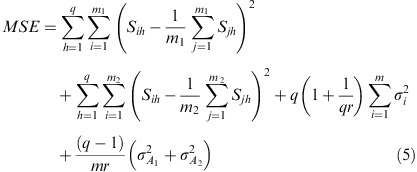Since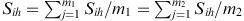, Equation (5) can be re-written as: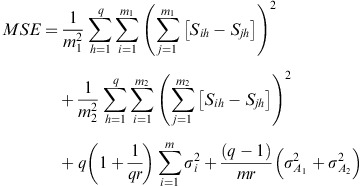which can be shown to be equal to: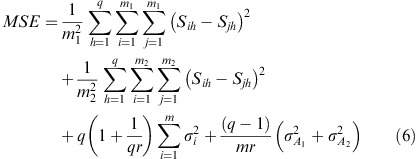Equation (4) translates directly to Equations (5) and (6) at the group level when we assume all items are independent. These theoretical results are exact.

When cross-correlations are present, the theoretical results are approximations. Equation (4) cannot be directly translated to Equations (5) and (6) at the group level because the way items are grouped has an impact on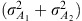. Due to the cross correlations, they are not the same as σ A 2. The effect of the approximations will be tested in an empirical analysis and the results are shown in a later section.

The distance metric that Bunn and Vassilopoulos (1993) and Vassilopoulos (1994) used was the Average Linkage between groups. Assume there are m1 series in the first group and m2 series in the second group. The grouping mechanism using the ‘average linkage between groups’ joins groups by minimising. It should be noted that this is not the same as the metric in Equation (6). The latter is based on differences of indices within groups, while the former is based on differences between groups.

Equations (4), (5) and (6) are MSEs based on universal application of the GSI method, which entails applying the GSI method to all series in the group. The total MSE for all items can be further reduced by applying GSI non-universally (and applying ISI to the other series). Our earlier research (Chen and Boylan, 2007) shows that even under the assumption of seasonal homogeneity within a group, it is not always better to apply GSI universally to all series. It is beneficial to apply GSI to noisy series as they ‘borrow strength’ from less noisy series. However, for ‘well behaved series’ (those with low noise), using ISI is better than GSI. In this current research, the assumption of seasonal homogeneity within groups is relaxed. We would expect further benefits of differential treatment of series (ie using ISI for some and GSI for others) when there is seasonal heterogeneity. This is examined later in the paper.

Conceptually, there are two possibilities when applying ISI and GSI methods. Suppose there are m series, which form a group. The first possibility is that GSI is applied to all of the series. The second is that GSI is calculated using all of the series, but applied only to some of the series. ISI is applied to the other series because they are less noisy. These series contribute to the formation of the seasonal group because their seasonal patterns are homogeneous to the group. However, because they are less noisy, it would be better to apply ISI to these series so that they do not ‘borrow weakness’ from the group.

To bring the applications of ISI and GSI together, we propose the following formula: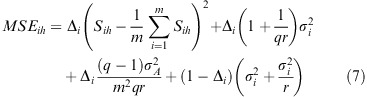where Δ I =1 if GSI is applied, 0 if ISI is applied.

Partitioning the group into two mutually exclusive groups with m1 and m2 number of series, the first part of the right hand side of the Equation (7) summed over i=1,…, m can be divided into two parts: one summed over i=1,…, m1 and the other over i=m1+1,…, m (which is the same as i=1,…, m2 because m1+m2=m):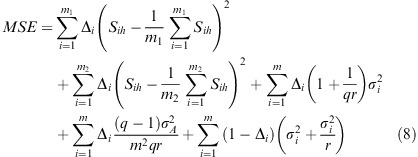In Equation (8), only the first two terms are affected by seasonal grouping; all others are not.

A general formulation of the problem is as follows, which is equivalent to a combinatorial optimisation problem:

Let Γ ij =1 if item i belongs to group j or 0 if i does not belong to a group, and Δ i =1 if GSI is applied or 0 if ISI is applied. i=1,…, m (m is the number of series) and j=1,…, n (n is the pre-determined number of seasonal groups).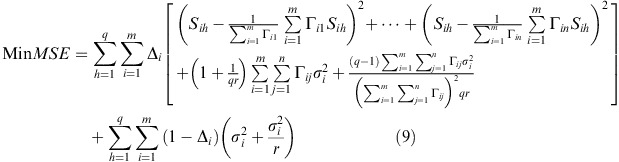such that:

each group must contain at least one item -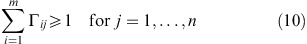each item must be in a group -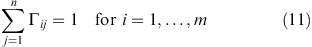Solving such a non-linear mixed integer optimisation problem is computationally challenging and time-consuming, depending on the number of items and number of seasonal groups. The complexity of this problem is mainly due to the interaction between the two sub-problems of (1) group formation and (2) decision to apply ISI or GSI at the level of the individual series. Therefore, we simplify the problem by considering the two sub-problems sequentially, ignoring the complex interaction between them. Therefore, we propose the following heuristic:

Assign the items into predetermined number of seasonal groups by minimising ∑h=1q(S ih −1/mi=1mS ih )2, ensuring that each item belongs to a group.

For each item i

Step 1::

assume Δ i =1 and calculate MSE using GSI

Step 2::

compare MSE using GSI with MSE using ISI

Step 3::

if MSE using ISI is less than MSE using GSI, then Δ i =0

Step 4::

repeat steps 2 and 3 until the total MSE is minimum.

This heuristic greatly simplifies the original problem and distinguishes the formation of seasonal groups and the application of GSI as two separate issues. The seasonal groups are defined by minimising the metric ∑h=1q(S ih −1/mi=1mS ih )2. Then the application of ISI and GSI is compared for each item. This means that some items may contribute to the formation of a seasonal group and the calculation of a GSI method, but ISI, rather than GSI, is applied to those series.

Thus, for the additive seasonal model, an approach has been developed that unifies the problems of group composition and forecasting method choice. This unification has been achieved through the common measure of MSE.

## 6. Seasonal grouping for the mixed model

When seasonality is multiplicative, there are two ways of calculating GSI: DGSI and WGSI. The MSE for a single item i by using both methods are expressed as follows (please refer to Appendix B):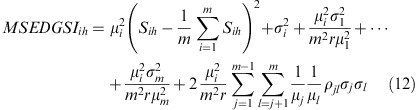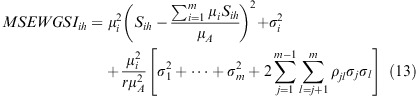These are the general expressions; currently as we assume no cross correlation, 2μ i 22rj=1m−1l=j+1m1/μ j 1/μ l ρ jl σ j σ l in Equation (12) and 2∑j=1m−1l=j+1mρ jl σ j σ l in Equation (13) is zero.

For the mixed model, a two-stage procedure for the minimisation of MSE is proposed, based on similar principles to the heuristic for the additive model, that is the formation of groups and application of ISI/GSI are treated separately. If we divide both sides of Equations (12) and (13) by μ i 2, they become: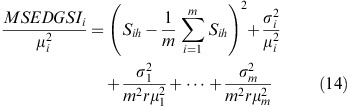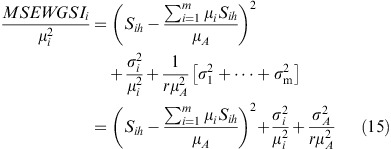When DGSI is used to calculate the MSE expression, the distance measure (S ih −1/mi=1mS ih )2 is the same as derived in the additive model. However, when WGSI is used, the distance measure (S ih −∑i=1mμiS ih /μ A )2 is different because WGSI is a weighted average of ISIs.

Again, at the group level, suppose there are m series and we wish to partition them into two mutually exclusive groups of m1 and m2 series (m1+m2=m). The right-hand side of Equation (14), summed over all seasons, becomes: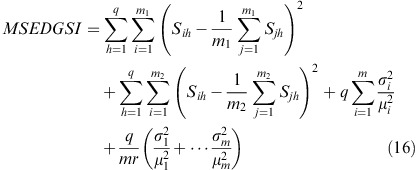Now, taking into account the potential application of either ISI or DGSI: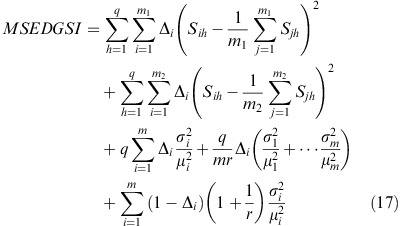The results for DGSI are still exact if the assumption of no cross correlation is maintained. It is important to note that the form of the Euclidean distance measure is identical to that of the additive model. Thus, the K-means clustering approach also applies to the application of DGSI (or ISI when appropriate) for the mixed model.

Moving on to the application of WGSI, the right-hand side of Equation (15) becomes: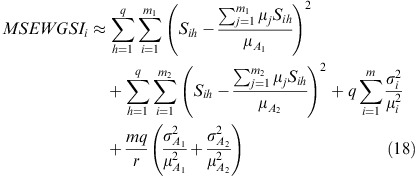This expression is an approximation because of the last term.

Now, taking into account the potential application of either ISI or GSI: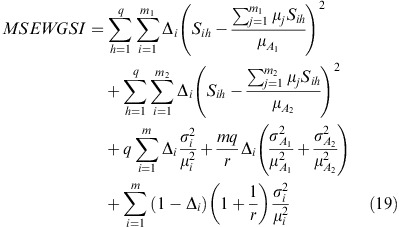The same heuristics can be applied to decide when to apply ISI and DGSI/WGSI in order to minimise MSE. When WGSI is applied, the distance metric for seasonal grouping is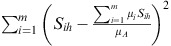.

It should be noted that this distance metric is not the same as for the additive model or for the mixed model (DGSI). Thus, the K-Means method should not be applied directly. Further research is currently being undertaken on adaptations of clustering methods for the mixed model (WGSI).

## 7. Empirical investigation

### 7.1. Experimental structure

In this section we analyse the empirical validity of some of the theoretical results presented thus far in the paper, with regard to both the formation of seasonally homogeneous groups and the issue of separating between the application of the ISI and GSI methods. We do so by means of experimentation with 218 real data series from the lighting industry. We should note that the same data set has also been utilised by Chen and Boylan (2008). The database contains the following information: (i) demand history recorded monthly for the period of October 1998 – September 2003 inclusive (60 monthly observations—5 years); (ii) the actual SKU grouping utilised by the company; the company has established seven product groups based on institutional knowledge. The actual groups have been made available to us but not the criteria upon which the grouping has taken place.

The 218 items are also grouped by using the K-means and the Average Linkage approach (utilising SPSS) to form seasonal groups. (In both cases the number of categories is forced to equal seven, ie the number of classes originally utilised by the company.) The former method is equivalent to the distance measure we derived from the additive model, and from using DGSI in the mixed model. The latter method is equivalent to the approach used by Bunn and Vassilopoulos (1993), and by Vassilopoulos (1994), discussed earlier in this paper.

Initial analysis was undertaken for both the additive and mixed models. Results, not reported here, showed that the differential strategy of using ISI for some series and GSI for others performs very well. However, the results relating to the universal application of GSI assuming additive seasonality (WGSI and DGSI are equivalent in this case) did not compare favourably with the mixed model. This was the case for all three approaches to grouping, namely company grouping, K-means and Average Linkage. For this reason, we have adopted a mixed model representation of the data.

As discussed in the previous section, further research is needed on clustering methods for the WGSI method when the mixed model is assumed. However, the K-means method has been found to be appropriate for the DGSI method. Consequently, we restrict the analysis of K-means clustering to the DGSI approach.

In practice, the sales of different items may well be correlated. For example, some products may be complementary to each other, while others may be substitutions. However, we still apply our original mixed model assuming no cross correlation. The empirical analysis will allow for an assessment of how good the theoretical approximations are when that assumption is violated.

We report universal application of ISI (all Δ i =0) and DGSI (all Δ i =1, based on the original company's grouping, the K-means and the Average Linkage methods). In the case of the GSI, such indices are calculated from, and applied in, each of the resulting groups (depending on the approach to grouping) separately. We also report the non-universal applications of ISI and DGSI, that is within a group DGSI is applied to some items and ISI to the rest based on which method results into a lower forecasting MSE across time for each series. We have considered two scenarios with regard to forecasting: (i) point forecasts—1, 3, 6 and 9 steps-ahead forecasts; (ii) cumulative forecasts over a forecast horizon of 3, 6 and 9 periods.

In more detail, the 5 years history is divided into two parts: (i) within sample that is equal to the first 4 years of data; (ii) out-of-sample, that contains the last 12 observations and is used for performance comparison purposes. In all cases, the within sample information is used to calculate the monthly ISI and the mean demand. Forecasting is then applied in a rolling overlapping fashion, which results in a dynamic simulation in the sense that we evaluate what would have happened if the particular methods had been used in practice by the company under concern. For example, consider the case of ISI. At the end of period 48, the mean demand (which is used as the deseasonalised demand) and the ISI calculated are used to produce four point forecasts (1, 3, 6 and 9 steps ahead) by multiplying the mean (deseasonalised) demand by the relevant seasonal factors. This information is also used to produce cumulative forecasts over a horizon of 3, 6 and 9 periods. At the end of period 49, the very first monthly observation (in period 1) is dropped and the 4-years data from November 1998 to October 2002 is used to calculate a new mean demand. The forecasting exercise is repeated and we continue in such a way until all data are exhausted. Please note that although 12 1-step-ahead forecasts are produced the number of the other point forecasts is not the same (there are 10 3-step-ahead forecasts, 7 6-step-ahead forecasts and 4 9-step-ahead forecasts). Similarly, for the cumulative forecasts over the forecast horizons considered.

To ensure consistency with earlier analysis conducted by Chen and Boylan (2008) errors are reported by using the symmetric Mean Absolute Percentage Error (sMAPE) measure, which is unit free. Absolute errors per period (or over an entire horizon in case of cumulative forecasts) are divided by the average of the actual demand in that period and the forecast produced for that period (or the average of cumulative demand over the horizon and the cumulative forecast for that time horizon) to form symmetric absolute percentage errors (sAPEs). These errors are then summarised across time per series by taking their arithmetic mean (sMAPE). An arithmetic mean is also used to average the sMAPEs per series across all 218 series. The advantages of the sMAPE over other relative error measures have recently been discussed in a comprehensive paper by Kolassa and Martin (2011).

In addition, and given that the theoretical results were derived based on the MSE, we have decided to employ this error metric as well. Squared errors are calculated per period (or horizon) and summarised across time per series by their arithmetic average (MSE). This particular measure is known to be heavily scale dependent and unduly influenced by the volume of the series. As such, MSEs are summarised across series by using a Relative Geometric summarisation (RGMSE). That is, the MSEs per series per method are summarised across all series with a geometric summarisation (GMSE). The RGMSE then is the ratio of the GMSEs related to any two methods. To standardise the presentation of the results all methods are compared against the ISI method (to be considered in the denominator). Values below 1 indicate performance in favour of the method under concern; values above 1 indicate a superior performance of the ISI method. Relative geometric summarisations have been shown (among others by Fildes 1992; Syntetos and Boylan, 2005) to be very robust. The theoretical properties and scale independent nature of the RGMSE make it a natural measure to be considered for the purposes of our experimentation. It allows the linkage of our empirical results to the theoretical analysis while a degree of ‘fairness’ is ensured for the comparison across series.

### 7.2. Empirical data and results

Before we discuss the empirical results and their analysis, it is important to provide an indication of the characteristics of the series used for the purposes of our investigation. We report four statistics that collectively capture the nature of the series and the degree (and nature) of the seasonality present in those series: (i) The mean demand per series across all 60 monthly observations; (ii) the ratio of the maximum over the minimum mean annual demand—the average annual demand is calculated for every SKU, across all 5 years of history, and the ratio of the maximum over the minimum average annual demand is reported as an indication of the scale differences present in the data; (iii) the minimum monthly seasonal index per series; (iv) the maximum monthly seasonal index per series. The distribution of these statistics then across all SKUs is presented based on some key quantities: minimum, 25th percentile, median, the 75th percentile and the maximum observation. The descriptive statistics are presented in Table 1 to the second decimal place.

The results indicate a great variation in terms of the underlying volumes of the series and the corresponding seasonal profiles.

The number of SKUs included in each of the seven categories as an outcome of the three grouping approaches considered in this paper (original company grouping, K-means, Average Linkage) is presented in Table 2. In the case of the company's approach the original grouping code and the description of the group are also provided. Please note that the Average Linkage approach results essentially in one very large group (almost identical to the entire dataset) and six very small groups. This is a common feature in its application; this method is known to build one very large group and many other much smaller.

The results for the sMAPE measure are summarised (across all series) in Table 3. The numbers presented are percentages (to the second decimal place). Please note that, following the theoretical analysis presented in this paper, the selection of the ISI or DGSI method in the two non-universal application approaches is based on the empirical MSE. This MSE-based selection is always used, regardless of weather the accuracy results are presented according to sMAPE or an MSE-related measure.

The first point that emerges from Table 3 is the consistent (and in some cases considerable) advantage of the non-universal application of the DGSI method over the universal application of it. This is true both for the K-means and the Average Linkage approach and this is the first empirical evidence to demonstrate a clear benefit of non-universal application of GSI. Further, the results provide some additional empirical evidence (to that already available in the literature) that grouping outperforms the ISI approach. (The comparison results between ISI and DGSI applied to all 218 SKUs may be found in Chen and Boylan, 2008.)

The second point is that, with universal application of the DGSI method, there is little difference between the forecast accuracy of the K-means and the Average Linkage approach (with the differences being overall in favour of K-means). Small differences in accuracy are also evident for non-universal application of methods (with the differences being overall in favour of Average Linkage).

More importantly, though, both the K-means approach and the Average Linkage (universal application) perform very similarly to the Company's grouping. Contrasting institutional knowledge with statistical applications indicates that there is little to choose between them. An important insight resulting from this comparison is that both statistical approaches may be safely applied in a real world context where no prior information is available that may be helpful for forming seasonal groups.

Finally, some similar findings to those reported in this paper were presented by Ouwehand et al (2005) with regard to the reduced accuracy as the forecast horizon increases and the increased accuracy for cumulative forecasts.

Next, we present the results related to the application of the RGMSE (Table 4). Results for all methods are presented in comparison with the ISI approach and thus the RGMSE for that method is simply 1. As aforementioned, numbers lower than 1, should be interpreted as an advantage in favour of the method under concern.

Overall, the results confirm our earlier findings on: (i) the superior performance of all grouping approaches to that of the ISI approach; (ii) the superiority of the non-universal application of the DGSI approach, under both the K-means and the Average Linkage method, over a universal application; (iii) the similar performance of the Average Linkage and K-means under both the universal and non-universal experimental scenarios.

One important issue that relates to the non-universal application of DGSI is the following: which are the characteristics of the series that determine when GSI or ISI perform better? To that end, we conducted a detailed analysis of the series where GSIs perform better than ISIs (and vice versa) in order to link the performance of methods to the underlying characteristics of the relevant series. For the additive model, Chen and Boylan (2007) found that the choice between ISI and the GSI depends on the variance of the deseasonalised series. Specifically, GSI is more accurate than ISI if: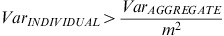where m is the number of the series contributing to the formation of the GSI. This is true forth for WGSI and DGSI.

For the mixed model, Chen and Boylan (op. cit.) found that the choice between ISI and the WGSI depends on the squared coefficient of variation of the deseasonalised series. That is, GSI is more accurate than ISI if: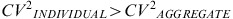For the mixed model and DGSI, a more complicated variance-based comparison rule was proposed.

As discussed in Section 5, collectively these results essentially tell us that if the individual series is less noisy than the group, ISI should be used. This means that those products that are less noisy than the group would only ‘borrow weakness’ from including noisier data. On the other hand, noisier series ‘borrow strength’ from less noisy series. If the ‘group’ is less noisy than the individual series, then it is better to use grouping methods. In other words, by inclusion of less noisy series in the group, noisier series would benefit.

Detailed analysis, not presented here, confirms the above findings. We have experimented both with the squared coefficient of variation and the variance of the deseasonalised series assessing the percentage of SKUs that behave according to theoretical expectations. That is, for every control parameter combination, the number of the series for which ISI/GSI is expected to perform better (based on either of the theoretical rules) was contrasted to the number of series on which ISI/GSI actually perform better. Very high percentages of series where theoretical expectations are sustained were reported (in many cases as high as 100%).

Before we close this section, we should also mention that the results on the cumulative forecasts are most important for the purposes of our analysis due to their inventory implications. In a stock control context, forecasts are required over a specific time horizon (either the lead time for continuous formulations or the lead time + review period for periodic inventory applications). Actual lead times have not been made available to us, so the forecast horizon may be interpreted as a lead time (+ review period) control parameter that has been assigned three reasonable values that collectively capture a wide range of real world scenarios (3, 6 and 9 periods). In addition, a forecast horizon of 1 period has been analysed, which can represent a unit review period and instantaneous supply.

## 8. Conclusions, implications and further research

This paper addressed two important research questions: how to form seasonally homogeneous groups and how to apply the ISI and GSI methods to improve forecasting accuracy at the item level. We have developed theoretical expressions to address these two issues and minimise MSE.

The expressions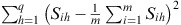andderived theoretically can be used as distance measures to define seasonal grouping. Previous researchers have recognised the grouping mechanism as a very important research issue but no other attempts have been so far to resolve this. The expressions we developed are theory-informed and are of a Euclidean form. The distance metrics are equivalent to the metric used in K-means clustering.

The clustering method employed by Bunn and Vassilopoulos (1993) is hierarchical in nature; it lacks any theoretical justification, although it provided satisfactory results in their empirical experiment. The same is true for the empirical investigation undertaken in our work. Although K-means was found to offer, overall, similar results to those of the Average Linkage approach, the theoretical basis associated with the former opens up opportunities for further developments in this area. Unifying the two problems of group composition and forecasting method choice, using the MSE measure, is an approach that may be extended to other forecasting models discussed in Section 2. The method may also be improved for the current models, by identifying better heuristic methods or by identifying the circumstances under which optimisation is feasible.

Our theoretical results are exact when assuming no cross correlation. When that assumption is relaxed, the theoretical findings can be used as an approximation. This was checked with the empirical analysis of 218 items, which were grouped into seven product families. First, our results showed that the groupings of both K-means and Average Linkage were competitive with the company's grouping, when seasonal methods were applied universally. Hence, there is some evidence that they may be safely applied in a real-world context where there is no prior information available for the formation of seasonal groups. Second, our results indicated that non-universal applications of ISI and GSI can improve forecasting accuracy compared to universal applications. This is the first paper to present empirical evidence to assess the effect on forecasting accuracy of switching from universal to non-universal application of seasonal index methods.

As part of our next steps of research, further simulations are to be conducted on the two clustering methods in order to understand more fully how various factors, including model parameters, affect forecasting performance. Experimentation with other datasets is also viewed as very important in order to expand the empirical knowledge base in the area of seasonal forecasting by grouping mechanisms. The effects of cross-correlations in the performance of GSI need to be further considered either by developing further the analytical work described in this paper to take this assumption into account or by further assessing the empirical robustness of our results in the presence of cross-correlations in real data. Moreover, the application of a heuristic procedure instead of the full optimisation model, when selecting between GSI and ISI, is to be assessed in more detail. This will involve the consideration of the trade-off between computational intensity of the optimisation solution and the effects on forecasting performance. Finally, the approach outlined in this paper will be extended to other seasonal models and forecasting methods.

## References

1. Bunn DW and Vassilopoulos AI (1993). Using group seasonal indices in multi-item short-term forecasting. International Journal of Forecasting 9 (4): 517–526.

2. Bunn DW and Vassilopoulos AI (1999). Comparison of seasonal estimation methods in multi-item short-term forecasting. International Journal of Forecasting 15 (4): 431–443.

3. Chen H and Boylan JE (2007). Use of individual and group seasonal indices in subaggregate demand forecasting. Journal of the Operational Research Society 58 (12): 1660–1671.

4. Chen H and Boylan JE (2008). Empirical evidence on individual, group and shrinkage seasonal indices. International Journal of Forecasting 24 (3): 525–534.

5. Chen H and Boylan JE (2009). Top-down, bottom-up and middle-out seasonal forecasting. Working Paper Series WP329/09, MaMS RI, University of Salford, UK.

6. Dalhart G (1974). Class seasonality – a new approach. American Production and Inventory Control Society 1974 Proceedings. Reprinted in Forecasting, 2nd edn., American Production and Inventory Control Society: Washington DC, pp. 11–16.

7. Dekker M, van Donselaar K and Ouwehand P (2004). How to use aggregation and combined forecasting to improve seasonal demand forecasts. International Journal of Production Economics 90 (2): 151–167.

8. Duncan G, Gorr W and Szczypula J (1993). Bayesian forecasting for seemingly unrelated time series: Application to local government revenue forecasting. Management Science 39 (3): 275–293.

9. Duncan G, Gorr W and Szczypula J (1998). Forecasting analogous time series. Working Paper 1998–4, Heinz School, Carnegie Mellon University, USA.

10. Fildes R (1992). The evaluation of extrapolative forecasting methods. International Journal of Forecasting 8 (1): 81–98.

11. Harvey AC (1989). Forecasting, Structural Time Series Models and the Kalman Filter. Cambridge University Press: Cambridge.

12. Kolassa S and Martin R (2011). Percentage errors can ruin your day (and rolling the dice shows how). Foresight: The International Journal of Applied Forecasting 23: 21–27.

13. Kim JH and Moosa IA (2005). Forecasting international tourist flows to Australia: A comparison between the direct and indirect methods. Tourism Management 26 (1): 69–78.

14. Miller DM and Williams D (2003). Shrinkage estimators of time series seasonal factors and their effect on forecasting accuracy. International Journal of Forecasting 19 (4): 669–684.

15. Ouwehand P, van Donselaar KH and de Kok AG (2005). The impact of the forecasting horizon when forecasting with group seasonal indices. Working paper 162, Eindhoven University of Technology, The Netherlands.

16. Rose DE (1977). Forecasting aggregates of independent ARIMA processes. Journal of Econometrics 5 (3): 323–345.

17. Stellwagen E (2004). Multiple-level forecasting. Presented at the Forecasting Summit, 27–29 September, Boston, USA.

18. Syntetos AA and Boylan JE (2005). The accuracy of intermittent demand estimates. International Journal of Forecasting 21 (2): 303–314.

19. Syntetos AA, Boylan JE and Disney SM (2009). Forecasting for inventory planning: A 50-year review. Journal of the Operational Research Society 60 (S1): S149–S160.

20. Vassilopoulos AI (1994). Development and evaluation of alternative methods for multi-item short-term business forecasting. Unpublished PhD thesis, London Business School, UK.

21. Withycombe R (1989). Forecasting with combined seasonal indices. International Journal of Forecasting 5 (4): 547–552.

## Acknowledgements

This research described in this paper has been funded by the Engineering and Physical Sciences Research Council (EPSRC, UK) grant number EP/G003858/1 (a project entitled: Using the grouping and shrinkage approaches to forecasting sub-aggregate level seasonal demand).

## Author information

Authors

### Corresponding author

Correspondence to J E Boylan.

## Appendices

### Appendix A

The additive model is specified as: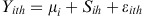The forecast for item i, the hth season in year r+1 using ISI is: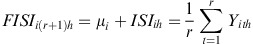The MSE of the forecast is: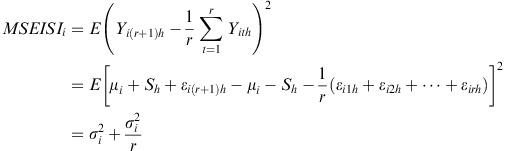Suppose we can find m items with similar seasonal patterns. It might be better to estimate their seasonality from the group.

The GSI estimator is given by: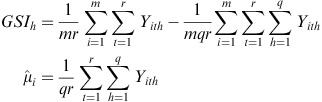Therefore, the forecast of item i, in the hth season in year r+1 is: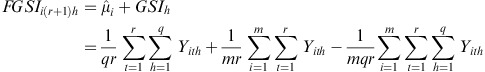The MSE of the forecast is as follows: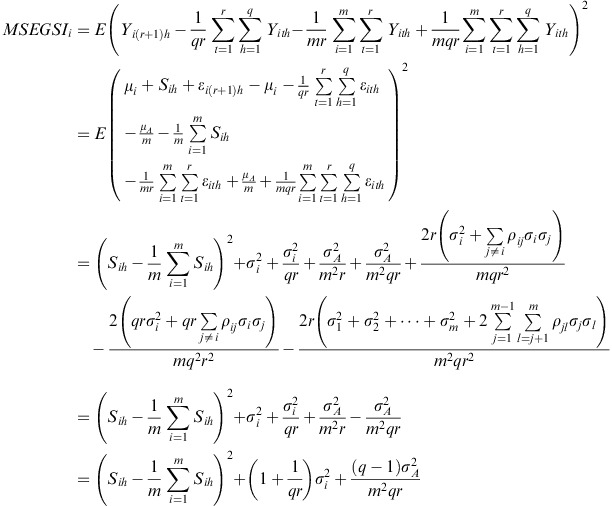MSEGSI i < MSEISI i if and only if: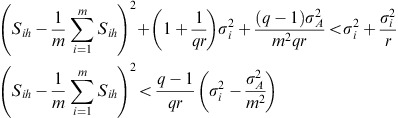### The mixed model

The mixed model is specified as: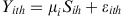In order to derive the rules for the mixed model, it is assumed that: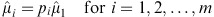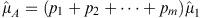where: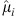is the estimate of μ i ,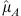is the estimate of μ A ,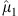is the estimate of the smallest mean value, and p i is the ratio between μ i and μ1 (p i =μ i /μ1).

The Mean Square Error (MSE) using ISI is the same as in the additive model.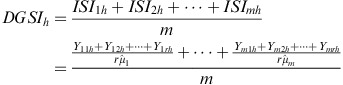The same forecast using DGSI is: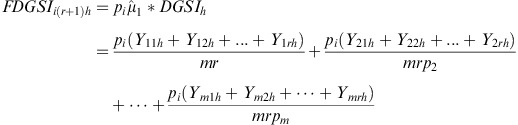The MSE is: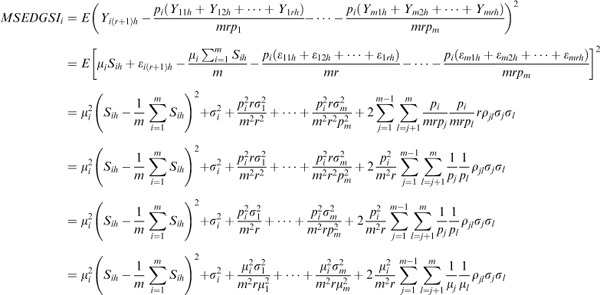MSEDGDI i < MSEISI i if and only if: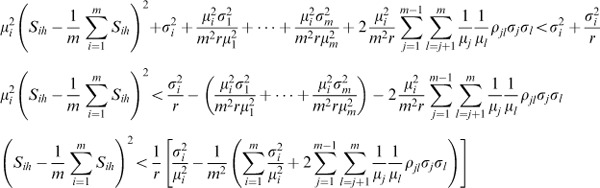For the WGSI method: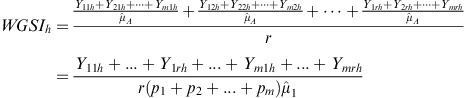The forecast of hth season in year r+1 for the ith item using WGSI is: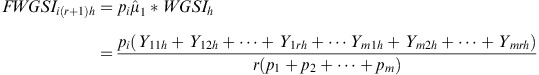The MSE is:MSEWGSI i < MSEISI i if and only if:## Rights and permissions

Reprints and Permissions

Boylan, J., Chen, H., Mohammadipour, M. et al. Formation of seasonal groups and application of seasonal indices. J Oper Res Soc 65, 227–241 (2014). https://doi.org/10.1057/jors.2012.126